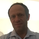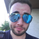35361 views
Stochastic Momentum Index (SMI) or Stoch MTM is used to find oversold and overbought zones. It also helps to figureout whether to enter short trade or long trade.

Red Shade in the Top indicates that the stock is oversold and the Green shade in the bottom indicates overbought.

Strategy:

Enter Long once the Overbought Zone ended and there's a crossover below -35.
Exit Long once the oversold zone is ended and there's a crossover.

Enter Short once the oversold zone is ended and there's a crossover above 35.
Exit Short once the Overbought Zone ended and there's a crossover.

Backup: Always use with another indicator because there will be multiple up and down movement in one Trend.
```//@version=2
//Stochastic Momentum Index
// Author: Surjith S M (India)
//Thanks UCSgears, lonestar108
study("Stochastics Momentum Index", shorttitle = "Stoch_MTM")
a = input(10, "Percent K Length")
b = input(3, "Percent D Length")
ob = input(40, "Overbought")
os = input(-40, "Oversold")
// Range Calculation
ll = lowest (low, a)
hh = highest (high, a)
diff = hh - ll
rdiff = close - (hh+ll)/2

avgrel = ema(ema(rdiff,b),b)
avgdiff = ema(ema(diff,b),b)
// SMI calculations
SMI = avgdiff != 0 ? (avgrel/(avgdiff/2)*100) : 0
SMIsignal = ema(SMI,b)
emasignal = ema(SMI, 10)

h0 = hline(40)
h1 = hline(-40)

//Color Definition for Stochastic Line
//col = SMI >= 40 ? green : SMI <= -40 ? red : black

plot(SMIsignal, title="Stochastic", style=line, color=black)

plot(emasignal, title="EMA", style=line, color=red)

level_40 = 40
level_40smi = SMIsignal > level_40 ? SMIsignal : level_40

level_m40 = -40
level_m40smi = SMIsignal < level_m40 ? SMIsignal : level_m40

p1 = plot(level_40)
p2 = plot(level_40smi)

p3 = plot(level_m40)
p4 = plot(level_m40smi)

fill(p1, p2, color=red, transp=40, title='OverSold')

fill(p3, p4, color=green, transp=40, title='OverBought')

```I think there is an error in your code. In Line 21, please check whether you need to use EMA function again. Plotting should be for SMI only. SMI signal calculation is superfluous as you have the EMA signal calculation in Line 22. Further the EMA signal for SMI the smoothing length is hard coded (10). This may not be apparent to the user.HI Surjit,

I am new to trading. I just started on trading view. I found your SMI indicator quite good. But just yesterday the earlier script was changed. I found a new indicator running on the chart with the same title. Could you please let me know how I could revert back to the earlier script. thanks Ram